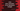# Java program to find the area and perimeter of an equilateral triangle## Java Program to find the area and perimeter of an equilateral Triangle :

In this tutorial, we will learn how to find the area and also perimeter of an equilateral triangle in Java.

All three sides of an equilateral triangle is equal and each angle is 60 degree. To find the area and perimeter, we only need the size of its one side.

Let’s check how to do it :

### Calculating the area :

Area of an equilateral triangle is = (√ 3 / 4) * side * side , where ‘side’ is the length of each side of the triangle. So, we need only the value of ‘side’ to calculate the area.

Let’s check the program :

``````import java.util.Scanner;

public class Main {

/**
* Utility functions
*/
static void println(String string) {
System.out.println(string);
}

public static void main(String[] args) {
Scanner sc = new Scanner(System.in); //1

println("Enter length of a side : ");
double side = sc.nextDouble(); //2

double area = (Math.sqrt(3) / 4) * side * side; //3

System.out.printf("Area of the triangle is %.2f",area); //4
}
}``````

### Explanation :

1. Create one ‘Scanner’ object
2. Take the input of the user as double using the Scanner class and save it in a variable called ‘side’
3. Calculate the area using formula ‘(√ 3 / 4) * side * side ‘ . For √ 3, use Math.sqrt(3).
4. The result we got on step 3 returns a double value. It will be something like 15.4456789 . But, do we want to print it as like ’15.44’ right ? So , we will convert this double to two decimal place as showing on step 4 above.

### Sample Output :

``````Enter length of a side :
6
Area of the triangle is 15.59

Enter length of a side :
10
Area of the triangle is 43.30``````

### Calculating the Perimeter :

Calculating the perimeter is much easier than calculating the area. Area of a triangle is the sum of all sides . For equilateral triangle, since its all sides are equal, the perimeter is (3 * length of one side) .

Java Program :

``````import java.util.Scanner;

public class Main {

/**
* Utility functions
*/
static void println(String string) {
System.out.println(string);
}

public static void main(String[] args) {
Scanner sc = new Scanner(System.in); //1

println("Enter length of a side : ");
double side = sc.nextDouble(); //2

double perimeter = 3 * side; //3

System.out.printf("Perimeter of the triangle is %.2f",perimeter); //4
}
}``````

### Explanation :

1. Create one ‘Scanner’ object
2. Take the input of the user as double using the Scanner class and save it in a variable called ‘side’
3. Calculate the area using formula 3 * side ‘.
4. Now print the result up to two decimal places

### Sample Output :

``````Enter length of a side :
12
Perimeter of the triangle is 36.00

Enter length of a side :
10
Perimeter of the triangle is 30.00``````